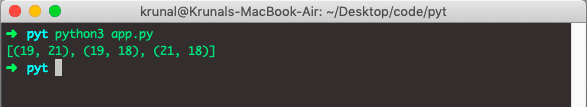# Python combinations: The Complete Guide

Python combinations are the selection of all or part of the set of objects without regard to the order in which the objects are selected. For example, suppose we have a set of three letters: A, B, and C. We might ask how many ways we can select two letters from that set. Each possible selection would be an example of a combination.

## Python combinations

To calculate combinations in Python, use the itertools.combinations() method. The itertools.combinations() method takes an iterator as an argument and returns all the possible combinations of elements in the iterator.

Python combinations are the same as Permutations except that No set will have the same elements as another. So, for example, we have a list of three items like [‘android’, ‘iOS’, ‘Symbian’ ], and if we choose only two elements and make a combination, then the total will be three combinations.

1. [‘android’, ‘iOS’]
2. [‘android’, ‘Symbian’]
3. [‘iOS’, ‘Symbian’]

### Example

Let’s take the example in Python Language and implement it.

```# app.py

from itertools import combinations

def combine(arr, s):
return list(combinations(arr, s))

array = [21, 18, 19]
set = 2
print(combine(array, set))```

In the above code, we have first imported the combinations object from the itertools module.

Then, we have defined a function called combine(), which will return the list of possible combinations. It takes an argument and creates a combination of those arguments.

In this example, we are making a list of 2 combinations.

See the output.## Calculating Combinations of Tuple In Python

To calculate the combinations of a tuple in Python, use the itertools.combinations() method. The combinations() method takes a tuple as an argument and returns all the possible combinations of elements of the tuple.

Let’s define a tuple and perform the combinations on the item of the tuple.

```# app.py

from itertools import combinations

def combine(tup, t):
return tuple(combinations(tup, t))

tup = (6, 29, 46)
set = 2
print(combine(tup, set))```

See the output.## Finding Combinations of Dictionary In Python

To calculate the combinations of a dictionary in Python, use the itertools.combinations() method. The combinations() method takes a dictionary as an argument and returns all the possible combinations of the dictionary elements.

Let’s define a dictionary and perform the combinations on the item of the tuple.

We will perform the combinations on the keys of the dictionary.

```# app.py

from itertools import combinations
def combine(dict, d):
return list(combinations(dict,d))
dictA = {
1: 19,
2: 21,
3: 18
}
set = 2
print(combine(dictA, set))```

See the output.We can perform the combinations on the values of the dictionary. See the following code.

```# app.py

from itertools import combinations
def combine(dict, d):
return list(combinations(dict,d))
dictA = {
1: 19,
2: 21,
3: 18
}
set = 2
print(combine(dictA.values(), set))```

See the output.That is for the Python combinations tutorial.

How to calculate mean in Python

How to calculate median in Python

How to calculate mode in Python

How to calculate variance in Python

How to calculate Standard Deviation in Python

### 1 thought on “Python combinations: The Complete Guide”

1.These are number what about alphabet
How we combine them